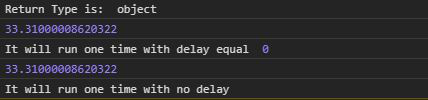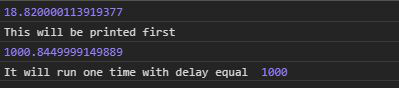Open in App
Not now

# D3.js timeout() Function

• Last Updated : 29 Jul, 2020

The d3.timeout() function in D3.js is used to automatically stop the function or the timer after a particular interval of time. It works same as setTimeOut() function in JavaScript.

Syntax:

```d3.timeout(callback, delay);
```

Parameters: This function accepts two parameters as mentioned above and described below:

• callback: It is the function to be stopped after a particular delay.
• delay: It is the time after which the function will be stopped.

Return Value: This function returns an object.

Below given are a few examples of the above function.

Example 1: When no delay is given.

## HTML

 ```<``html` `lang``=``"en"``>`` ` `<``head``>``    ``<``meta` `charset``=``"UTF-8"``>``    ``<``meta` `name``=``"viewport"` `content``=``    ``"width=device-width, initial-scale=1.0"``>```` ` `<``body``>``    ````    ``<``script` `type``=``"text/javascript"` `        ``src``=``"https://d3js.org/d3.v4.min.js"``>``    ````     ` `    ``<``script``>``        ``let delay = 0``        ``let func = function (e) {``            ``console.log(e);``            ``console.log("It will run one time"``                ``+ " with delay equal ", delay);``        ``}``        ``var timer = d3.timeout(func, delay);`` ` `        ``func = function (e) {``            ``console.log(e);``            ``console.log(``                ``"It will run one time with no delay");``        ``}``        ``var timer = d3.timeout(func);``        ``console.log("Return Type is: ", typeof timer);``    `````` ` ``

Output:Example 2: When the delay is given.

## HTML

 ```<``html` `lang``=``"en"``>`` ` `<``head``>``    ``<``meta` `charset``=``"UTF-8"``>``    ``<``meta` `name``=``"viewport"` `content``=``        ``"width=device-width, initial-scale=1.0"``>```` ` `<``body``>``    ````    ``<``script` `type``=``"text/javascript"` `        ``src``=``"https://d3js.org/d3.v4.min.js"``>``    ````     ` `    ``<``script``>``        ``let delay = 1000``        ``let func = function (e) {``            ``console.log(e);``            ``console.log("It will run one time"``                ``+ " with delay equal ", delay);``        ``}``        ``var timer = d3.timeout(func, delay);`` ` `        ``func = function (e) {``            ``console.log(e);``            ``console.log("This will be printed first");``        ``}``        ``var timer = d3.timeout(func);``    `````` ` ``

Output:My Personal Notes arrow_drop_up